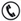Danh mục
Dịch vụ
Hỗ trợ trực tuyến

### Mr: MạnhMr: Thuận

# Quy trình thiết kế lắp đặt trạm Gas (LPG)

KIẾN THỨC CHUNG VỀ LPG

 You can convert: LPG kg to litres (1kg = 1.96L) or litres to kg (1L = 0.51kg) *LPG kg to m³ (1kg = 0.53m³) or m³ to kg (1m³ = 1.89kg) *LPG litres to m³ (1L = 0.27m³) or m³ to litres (1m³ = 3.70L) LPG MJ to kWh (1MJ = 0.278kWh) or kWh to MJ (1kWh = 3.6 MJ) LPG litres to MJ and vice versa (1L = 25MJ) or (1MJ = 0.042L) LPG kg to MJ and vice versa (1kg = 49MJ) or (1MJ = 0.02kg) LPG litres to kWh and vice versa (1L = 6.9kWh) or (1kWh = 0.145L) LPG kg to kWh and vice versa (1kg = 13.6kWh) or (1kWh = 0.074kg) and all other combinations
Các bài viết khác
Dự án tiêu biểu